Next: Monte-Carlo integration Up: Monte-Carlo methods Previous: Random numbers

## Distribution functions

Let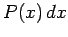represent the probability of finding the random variablein the intervalto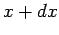. Here,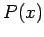is termed a probability density. Note that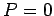corresponds to no chance, whereas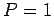corresponds to certainty. Since it is certain that the value oflies in the range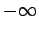to, probability densities are subject to the normalizing constraint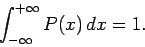(315)

Suppose that we wish to construct a random variablewhich is uniformly distributed in the range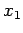to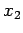. In other words, the probability density ofis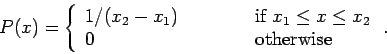(316)

Such a variable is constructed as follows
x = x1 + (x2 - x1) * double (random ()) / double (RANDMAX);


There are two basic methods of constructing non-uniformly distributed random variables: i.e., the transformation method and the rejection method. We shall examine each of these methods in turn.

Let us first consider the transformation method. Let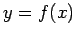, where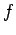is a known function, andis a random variable. Suppose that the probability density ofis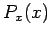. What is the probability density,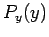, of? Our basic rule is the conservation of probability: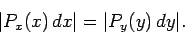(317)

In other words, the probability of findingin the intervaltois the same as the probability of findingin the intervalto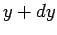. It follows that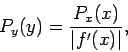(318)

where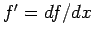.

For example, consider the Poisson distribution: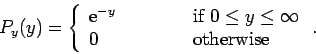(319)

Let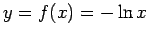, so that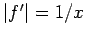. Suppose that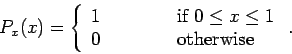(320)

It follows that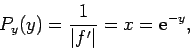(321)

with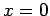corresponding to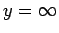, and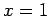corresponding to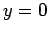. We conclude that if
x = double (random ()) / double (RANDMAX);
y = - log (x);

then y is distributed according to the Poisson distribution.

The transformation method requires a differentiable probability distribution function. This is not always practical. In such cases, we can use the rejection method instead.

Suppose that we desire a random variabledistributed with densityin the range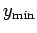to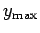. Let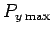be the maximum value of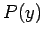in this range (see Fig. 95). The rejection method is as follows. The variableis sampled randomly in the rangeto. For each value ofwe first evaluate. We next generate a random numberwhich is uniformly distributed in the range 0 to. Finally, if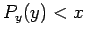then we reject thevalue; otherwise, we keep it. If this prescription is followed thenwill be distributed according to.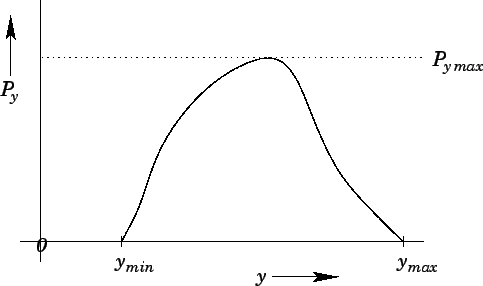As an example, consider the Gaussian distribution: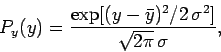(322)

where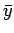is the mean value of, and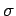is the standard deviation. Let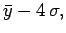(323)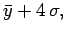(324)

since there is a negligible chance thatlies more than 4 standard deviations from its mean value. It follows that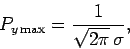(325)

with the maximum occurring at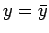. The function listed below employs the rejection method to return a random variable distributed according to a Gaussian distribution with mean mean and standard deviation sigma:
// gaussian.cpp

// Function to return random variable distributed
// according to Gaussian distribution with mean mean
// and standard deviation sigma.

#define RANDMAX 2147483646

int random (int = 0);

double gaussian (double mean, double sigma)
{
double ymin = mean - 4. * sigma;
double ymax = mean + 4. * sigma;
double Pymax = 1. / sqrt (2. * M_PI) / sigma;

// Calculate random value uniformly distributed
//  in range ymin to ymax
double y = ymin + (ymax - ymin) * double (random ()) / double (RANDMAX);

// Calculate Py
double Py = exp (- (y - mean) * (y - mean) / 2. / sigma / sigma) /
sqrt (2. * M_PI) / sigma;

// Calculate random value uniformly distributed in range 0 to Pymax
double x = Pymax * double (random ()) / double (RANDMAX);

// If x > Py reject value and recalculate
if (x > Py) return gaussian (mean, sigma);
else return y;
}

Figure 96 illustrates the performance of the above function. It can be seen that the function successfully returns a random value distributed according to the Gaussian distribution.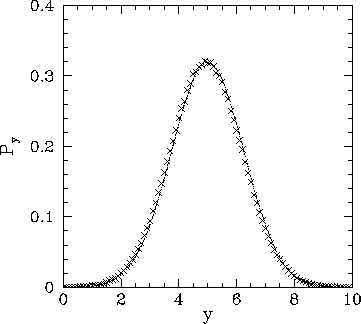Next: Monte-Carlo integration Up: Monte-Carlo methods Previous: Random numbers
Richard Fitzpatrick 2006-03-29